• max(): max(a)求矩阵每一列的最大值； find(): find (a == Elem)查找Elem在矩阵中a中的行数和列数 eg： 矩阵a = [4,5,6,9,4;8,5,21,2,65;36,78,59,3,5;7,8,9,6,5] 4✖5 查找出最大值是78； 用find函数找到最大值...
已知矩阵a ;
max():  max(a)求矩阵每一列的最大值；
find():  find (a == Elem)查找Elem在矩阵中a中的行数和列数
eg：
矩阵a = [4,5,6,9,4;8,5,21,2,65;36,78,59,3,5;7,8,9,6,5] 4✖5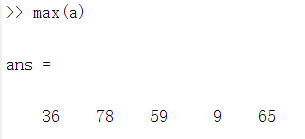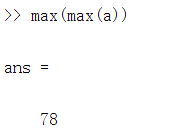查找出最大值是78；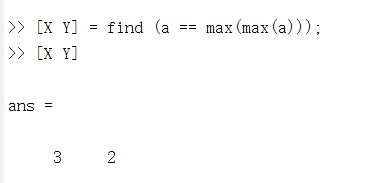用find函数找到最大值的位置
总代码
>> a = [4,5,6,9,4;8,5,21,2,65;36,78,59,3,5;7,8,9,6,5];
>> max(a)

ans =

36    78    59     9    65

>> max(max(a))

ans =

78

>> [X Y] = find (a == max(max(a)));
>> [X Y]

ans =

3     2

>>



展开全文• 假设矩阵为x，则最大值为max(max(x))。 所在位置为：[rows,cols]=... [Y,I]=max(M,[],2), 在第2维方向上取最大值，也就是最大值，结果存在Y里，I里存最大值的列位置。 >> M=magic(3) M = 8 1 6 3 5 7 4 9
 假设矩阵为x，则最大值为max(max(x))。 所在位置为：[rows,cols]=find(x==max(max(x)))。 嗯，这样就可以啦。

用find和max命令，多用help命令。
[Y,I]=max(M,[],2), 在第2维方向上取最大值，也就是每行最大值，结果存在Y里，I里存的是每行最大值的列位置。 >> M=magic(3) M = 8 1 6 3 5 7 4 9 2 >> [Y,I]=max(M,[],2) Y = 8 7 9 I = 1 3 2
max求一个数组的最大元素函数。用法C = max(A)返回一个数组各不同维中的最大元素。如果A是一个向量，max(A)返回A中的最大元素。如果A是一个矩阵，max(A)将A的每一列作为一个向量，返回一行向量包含了每一列的最大元素。如果A是多为数组，max(A) treats the values along the first non-singleton dimension as vectors, returning the maximum value of each vector. C = max(A,B)返回一个和A和B同大小的数组，其中的元素是从A或B中取出的最大元素。C = max(A,[],dim)返回A中有dim指定的维数范围中的最大值。[C,I] = max(...)找到A中那些最大值的索引位置，将他们放在向量I中返回。如果这里有多个相同最大值时，返回的将是第一个的索引。-----------------------------------------------max Maximum elements of an array SyntaxC = max(A)C = max(A,B)C = max(A,[],dim)[C,I] = max(...)DescriptionC = max(A) returns the largest elements along different dimensions of an array. If A is a vector, max(A) returns the largest element in A. If A is a matrix, max(A) treats the columns of A as vectors, returning a row vector containing the maximum element from each column. If A is a multidimensional array, max(A) treats the values along the first non-singleton dimension as vectors, returning the maximum value of each vector. C = max(A,B) returns an array the same size as A and B with the largest elements taken from A or B. C = max(A,[],dim) returns the largest elements along the dimension of A specified by scalar dim. For example, max(A,[],1) produces the maximum values along the first dimension (the rows) of A. [C,I] = max(...) finds the indices of the maximum values of A, and returns them in output vector I. If there are several identical maximum values, the index of the first one found is returned. RemarksFor complex input A, max returns the complex number with the largest complex modulus (magnitude), computed with max(abs(A)), and ignores the phase angle, angle(A). The max function ignores NaNs.

展开全文• %求每一的最大值 end c=max(b) %求得最大值 [i,j]=find(a==c)%i，j分别代表行和坐标 要想做好这个题，首先熟练知道matlab中max的用法以及了解matlab中关于矩阵行的操作 以及通过做这个题了解了matlab中find函数...
问题一：
有一个45 矩阵,用matlab编程求出其最大值及其所处的位置.
二话不说，上代码
clc;
clear;
a=fix(100rand(4,5))% 随机生成1–100的4X5的整数矩阵
for i=1:4
b=max(a(i,:));%求每一行的最大值
end
c=max(b) %求得最大值
[i,j]=find(a==c)%i，j分别代表行和列坐标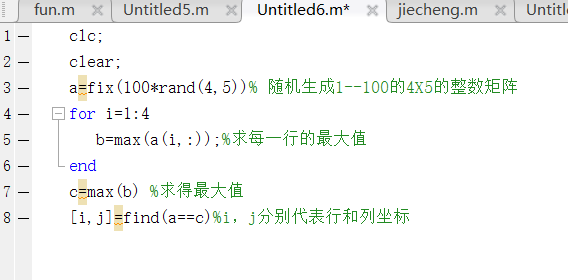要想做好这个题，首先熟练知道matlab中max的用法以及了解matlab中关于矩阵行的操作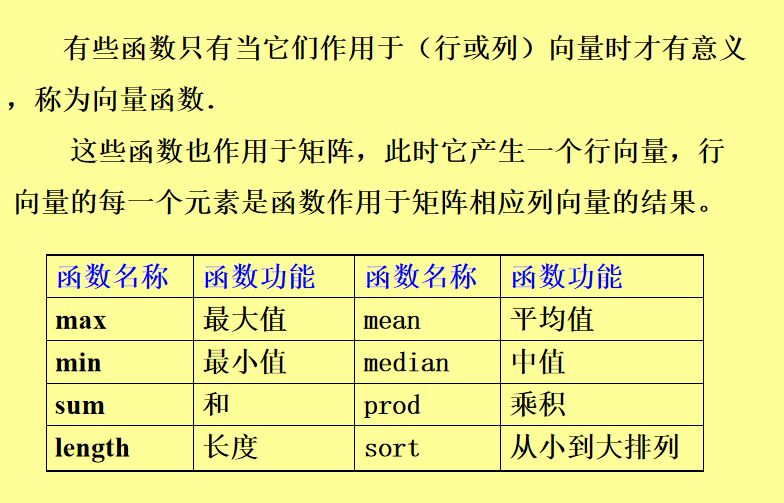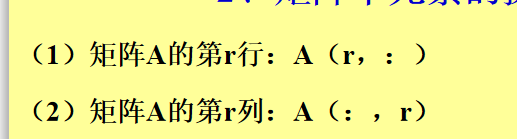以及通过做这个题了解了matlab中find函数的用法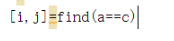i，j分别代表行和列坐标
所以最后结果就是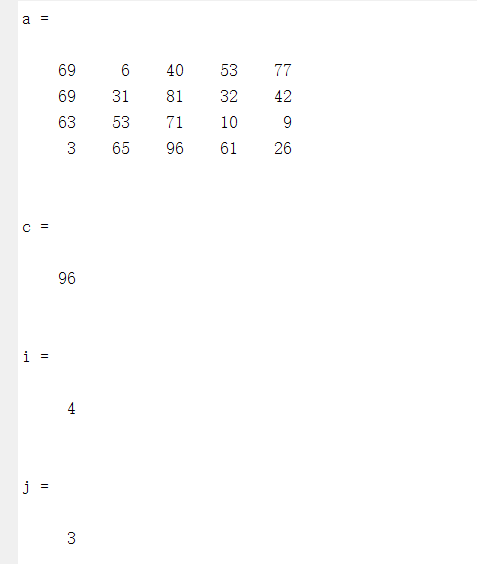最后补充几点关于矩阵元素的操作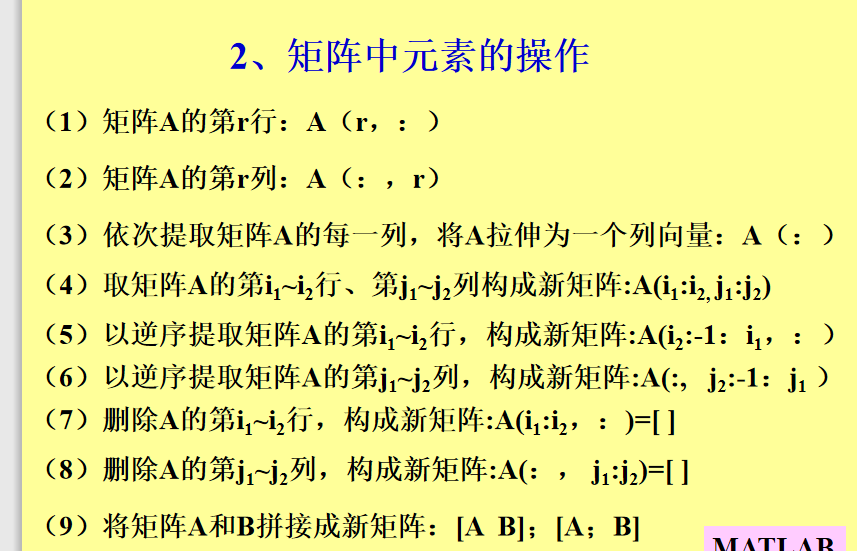展开全文• 矩阵x的每一列存储的是相应的特征向量，所以最后一列就是矩阵A的最大特征所对应的特征向量 实例运用： 1、先创建一个矩阵 2、调用eig()函数，所得矩阵x就是矩阵A的特征向量 3、调用diag()函数，所得结果就是...
在MATLAB语言中，求矩阵的特征值和特征向量需要用到两个函数：eig()、diag()
diag():可生成一个对角矩阵
调用eig函数的格式为：

[x,y]=eig(A)

其中矩阵y的对角线元素存储的是A的所有特征值，且从小到大排列；而矩阵x的每一列存储的是相应的特征向量，所以最后一列就是矩阵A的最大特征值所对应的特征向量
实例运用：
1、先创建一个矩阵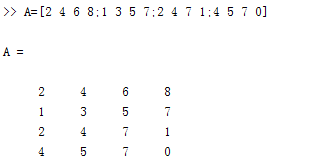2、调用eig()函数，所得矩阵x就是矩阵A的特征向量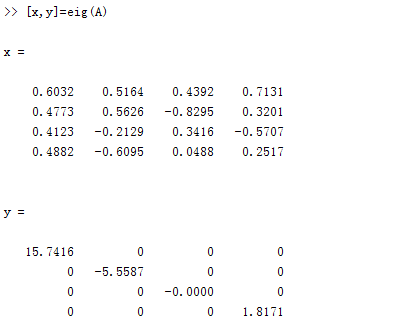3、调用diag()函数，所得结果就是矩阵A的特征值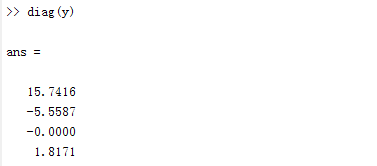展开全文线性代数
• 默认返回每列的最大值，向量 max(a,2) a中个元素与2取大的，返回矩阵 max(a,[],dim) dim表示维度，1为行中最大的，2为列中最大的… 3、取整函数 ceil(a) 向上取整 floor(a) 向下取整 fix...函数
• 1、矩阵第 i 行的元素满足：aij=a10+(j-1)*i 或者 aij=a10-(j-1)*i （即每一行相邻元素的间隔都为 i 或都为 -i）; 2、矩阵的元素都为正整数；...求最小的正整数M，M是满足条件的矩阵中元素的最大值
• .矩阵分析 1.向量和范数运算 ...矩阵的1阶范数为矩阵每列之和的最大值，2阶范数为矩阵的最大奇异值，即A’A矩阵的最大特征值的开平方，∞阶范数为矩阵每行之和的最大值，F-范数为即矩阵元素绝...
• [u,v]=find(a==max(a(:,i)))%本意是找到a中每列的最大值，并将其用255代替 a(u,v)=255 end >>a = 3 4 80 255 255 255 255 255 255 解释：出现此结果的原因：find中用的是a,每一次循环会找到可能几个跟max(a(:...
• 若已知矩阵为A，则[V,D]=eig(A)，其中对角阵D对角元素为A特征，而矩阵D的每一列向量为其所对应特征向量.A = 0.3132 0.3566 0.2545 0.2579 0.2063 -0.0897 0.2913 0.1888 0.4392 0.1470 0.0845 0...null
• (2) [Y,U]=min(A)：返回行向量Y和U，Y向量记录A的每列的最小值，U向量记录列最小值的行号。 (3) min(A,[],dim)：dim取1或2。dim取1时，该函数和min(A)完全相同；dim取2时，该函数返回个列向量，其第i个元素是A...
• sum求和操作max求最大值操作sum：求和操作sum(A)：矩阵A按列向求和(每一列求和)，结果为一个行向量...max：最大元素操作仅返回最大值情况max(A)：矩阵A按列向求最大值(每一列的最大值)，结果为一个行向量max(A,[],2)...
• 1、max 是把每列的最大值打印出来 2、max(max(A)) 是把整个矩阵元素的最大值打印出来 3、min(A) 是把列的最小值打印出来 4、sum(A) 是把列的加和求出来 5、sort(A) 是把列从小排到大，显示出来 6、...
• 2014年7月8日 matlab矩阵是按列存储的，对一个二维矩阵A使用max函数 max（A）是求A中每一列的最大值，并以行向量的形式表示 max（A（：））是求A中所有元素中的最大值
• Matlab中max函数在矩阵中求...如果A是一个矩阵，max(A)将A的每一列作为一个向量，返回一个行向量,向量的第i个元素是矩阵A的第i列上的最大值。如果A是多维数组，max(A) treats the values along the first non-single...
• 矩阵每列的最大最小值 如果a是个m行n列的矩阵。max(a)则求列中的最大值，返回值为1行n列数。 如下程序： 求最大值的运行结果如下： 求最小值： 求矩阵中的最大最小值 我们可以看到用矩阵...max
• 3. 矩阵每一列的最大值max（A） 4. 矩阵每一列的最小值min（A） 5. 矩阵每一列的和sum（A），若为向量则是所有元素的和 6. 向下取整 floor（A），对复数来说是分别对实部和虚部进行运算 7. 向上取整ceil（A），对...
• ## matlab max/min函数用法

万次阅读 多人点赞 2018-06-04 10:06:58
matlab中的max/min函数 求矩阵的最大值和最小值 ...(2) [Y,U]=max(A)：返回行向量Y和U，Y向量记录A的每列的最大值，U向量记录列最大值的行号。 (3) max(A,[],dim)：dim取1或2。dim取1时，该...
• 如果A为矩阵，则max(A)是包含每一列的最大值的行向量。 M= max(A,[],dim)返回维度dim上的最大元素。例如，如果A为矩阵，max(A,[],1)=max(A)返回每一列的最大值的列向量，max(A,[],2)是包含每一行的最大值的列...
• 当A是一个列向量时候，返回一个最大值，在此不在赘述。 当Amxn是一个矩阵的时候，有以下几种情况： ...③ E = max(A,[],2)，返回每一列的最大值，即1xm的列向量 ④ F = max(A,8)，当元素小于8，用8填充 ⑤ ...
• 数据统计分析 求最大元素与最小元素 参数为向量时： y=max(X): 返回向量X的最大值存入y...[Y,U]=max(A):返回行向量Y和U，Y向量记录A的每列的最大值, U向量记录列最大值元素的行号。 max(A,I,dim): dim取1或2。dim取数据分析
• Matlab中max函数在矩阵中求函数...（2）[Y,U]=max(A)：返回行向量Y和U，Y向量记录A的每列的最大值，U向量记录列最大值的行号。 （3）max(A,[],dim)：返回A中有dim指定的维数范围中的最大值。 dim取1或2。 dim取1时字符串
• 细说matlab中的max函数 max函数的几种形式 (1)max(a) (2)max(a,b) (3)max(a,[],dim) (4)[C,I]=max(a) (5)[C,I]=max(a,[],dim) 二举例...4,5,6]max(a)的意思就是找出矩阵每列的最大值 本例中max(a)=[4,5,6] (2)max(a,
• (2) [Y,U]=max(A)：返回行向量Y和U，Y向量记录A的每列的最大值，U向量记录列最大值的行号。 (3) max(A,[],dim)：dim取1或2。dim取1时，该函数和max(A)完全相同；dim取2时，该函数返回个列向量，其第i个...max
• ## matlab中的 MAX函数

千次阅读 2016-10-13 20:07:01
matlab中的 MAX函数的几种形式 　(1)max(a) 　(2)max(a,b) 　(3)max(a,[],dim) 　(4)[C,I]=max(a) 　(5)[C,I]=max(a,[],dim) ...4,5,6]，max(a)的意思就是找出矩阵每列的最大值， 本例中：max(a)=[4,函数
• Matlab中max函数在矩阵中求...如果A是一个矩阵，max(A)将A的每一列作为一个向量，返回一个行向量,向量的第i个元素是矩阵A的第i列上的最大值。 如果A是多维数组，max(A) treats the values along the first non-...
• %max在把矩阵每列的最大值找到，并组成个单行的数组，转置一下就会行转换为列，再max就求个最大的值，如果不转置，只能求出每列的最大值。 f = 1 - I/maxvalue; %为什么要用1去减？ Image1=f; [xsize ...
• ## Matlab函数

千次阅读 2016-08-07 01:03:31
MAX: 当A是一个列向量时候，返回一个最大值，在此不在赘述。 当Amxn是一个矩阵的时候，有以下几种情况： ...③ E = max(A,[],2)，返回每一列的最大值，即1xm的列向量 ④ F = max(A,8)，当元素小于8，用8填充 ⑤数学
• (2) [Y,U]=max(A)：返回行向量Y和U，Y向量记录A的每列的最大值，U向量记录列最大值的行号。 (3) max(A,[],dim)：dim取1或2。dim取1时，该函数和max(A)完全相同；dim取2时，该函数返回个列向量，其第i个元素是A...
• 矩阵A的最大值的函数有3种调用格式，分别是： 1. max(A)：返回一个行向量，向量的...如果A是一个矩阵，max(A)将A的每一列作为一个向量，返回一行向量包含了每一列的最大元素。 如果A是多为数组，max(A) treats t...max
• 数据分析与多项式计算 数据统计分析 求最大元素和最小元素 ...max(a)：返回个行向量，向量的第i个元素是矩阵a的第i的最大值 [y,u] = max(a)：返回行向量y和u，y向量记录a的每列最大值，u向量记录每列最大数学建模 数据挖掘# matlab矩阵每一列的最大值matlab 订阅